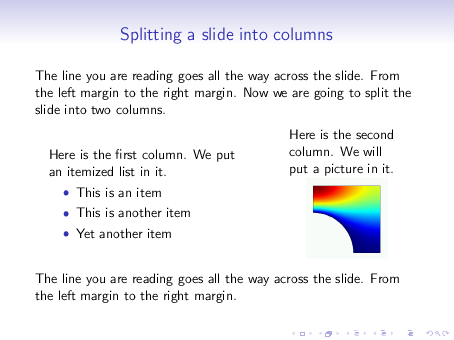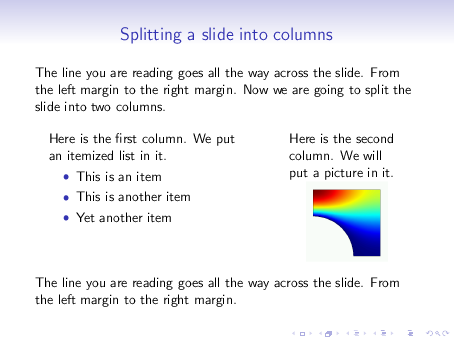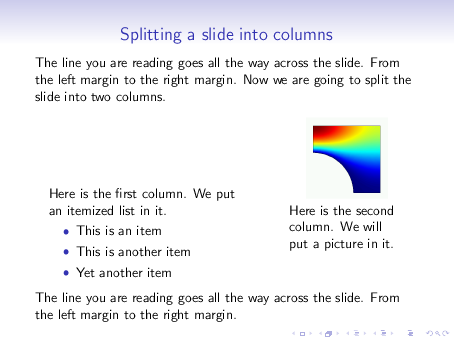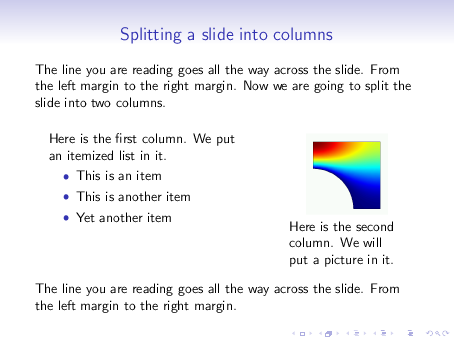# 18  Splitting a slide into columns

Beamer’s columns environment provides an easy way to split a slide vertically into columns. This is particularly useful in positioning figures in a slide or creating multi-column itemized lists.

The following example shows how.

% columns-demo1.tex
\documentclass{beamer}
\usetheme{Singapore}
\begin{document}

\begin{frame}{Splitting a slide into columns}

The line you are reading goes all the way across the slide.
From the left margin to the right margin.  Now we are going
to split the slide into two columns.
\bigskip

\begin{columns}
\begin{column}{0.5\textwidth}
Here is the first column.  We put an itemized list in it.
\begin{itemize}
\item This is an item
\item This is another item
\item Yet another item
\end{itemize}
\end{column}

\begin{column}{0.3\textwidth}
Here is the second column.  We will put a picture in it.
\centerline{\includegraphics[width=0.7\textwidth]{image2.png}}
\end{column}
\end{columns}
\bigskip

The line you are reading goes all the way across the slide.
From the left margin to the right margin.

\end{frame}

\end{document}


The input file above produces the following slide:Remark: To split a slide into three or more columns, add any number of \begin{column}...\end{column} as needed. Just make sure that the sum of their widths does not exceed the slide width, that is \textwidth.

Remark: Within each column, the variable \textwidth is redefined to refer to that column’s width. For instance, in the sample shown above, the width of the image is set to 0.7\textwidth which means 0.7 times the width of the column that contains the image.

Remark: I find it easier giving widths of columns in terms of fractions of \textwidth. If you wish, however, you may specify absolute widths, such as: \begin{column}{30mm}. For this, you should know that the overall size of a Beamer slide is 128mm x 96mm.

## Vertical alignment

Observe that in the sample slide shown above, the vertical mid-points of the two columns are horizontally aligned. We say the columns are center-aligned, for short.

The option [t] to the columns environment, as in \begin{columns}[t], makes the columns top-aligned.

Other options are [b] for bottom-alignment and [c] for center-alignment (which is the default).

In the following example the columns are top-aligned:

% columns-demo2.tex
\documentclass{beamer}
\usetheme{Singapore}
\begin{document}

\begin{frame}{Splitting a slide into columns}

The line you are reading goes all the way across the slide.
From the left margin to the right margin.  Now we are going
to split the slide into two columns.
\bigskip

\begin{columns}[t]
\begin{column}{0.5\textwidth}
Here is the first column.  We put an itemized list in it.
\begin{itemize}
\item This is an item
\item This is another item
\item Yet another item
\end{itemize}
\end{column}

\begin{column}{0.3\textwidth}
Here is the second column.  We will put a picture in it.
\centerline{\includegraphics[width=0.7\textwidth]{image2.png}}
\end{column}
\end{columns}
\bigskip

The line you are reading goes all the way across the slide.
From the left margin to the right margin.

\end{frame}

\end{document}## Vertical alignment with images

Sometimes you may find out that the option [t] for top-alignment gives unexpected results. The following example shows one such case. The only difference between this and the previous example is that I have interchanged the image and text in the second column.

% columns-demo3.tex
\documentclass{beamer}
\usetheme{Singapore}
\begin{document}

\begin{frame}{Splitting a slide into columns}

The line you are reading goes all the way across the slide.
From the left margin to the right margin.  Now we are going
to split the slide into two columns.
\bigskip

\begin{columns}[t]
\begin{column}{0.5\textwidth}
Here is the first column.  We put an itemized list in it.
\begin{itemize}
\item This is an item
\item This is another item
\item Yet another item
\end{itemize}
\end{column}

\begin{column}{0.3\textwidth}
\centerline{\includegraphics[width=0.7\textwidth]{image2.png}}
Here is the second column.  We will put a picture in it.
\end{column}
\end{columns}
\bigskip

The line you are reading goes all the way across the slide.
From the left margin to the right margin.

\end{frame}

\end{document}As you see, the columns are not top-aligned at all!

What is happening here is that the bottom of the image is being taken as the image’s reference point. Therefore the bottom of the image is being aligned with the top of the first column. This is not what we want!

We want the top of the image to be taken as the reference point, so that the top of the image is aligned with the top of the first column. To achieve this, Beamer provides the [T] alignment option. The following example shows the result.

% columns-demo4.tex
\documentclass{beamer}
\usetheme{Singapore}
\begin{document}

\begin{frame}{Splitting a slide into columns}

The line you are reading goes all the way across the slide.
From the left margin to the right margin.  Now we are going
to split the slide into two columns.
\bigskip

\begin{columns}[T]
\begin{column}{0.5\textwidth}
Here is the first column.  We put an itemized list in it.
\begin{itemize}
\item This is an item
\item This is another item
\item Yet another item
\end{itemize}
\end{column}

\begin{column}{0.3\textwidth}
\centerline{\includegraphics[width=0.7\textwidth]{image2.png}}
Here is the second column.  We will put a picture in it.
\end{column}
\end{columns}
\bigskip

The line you are reading goes all the way across the slide.
From the left margin to the right margin.

\end{frame}

\end{document}